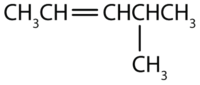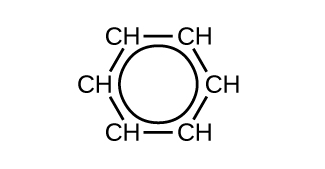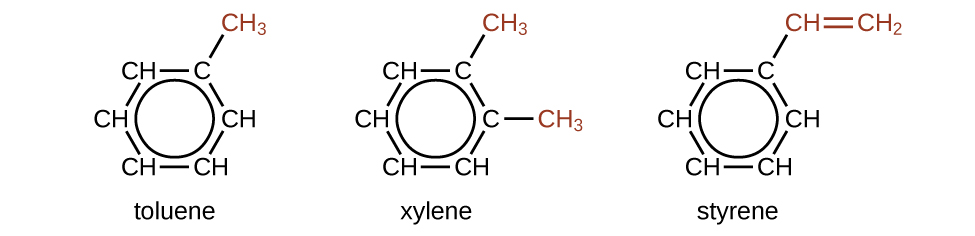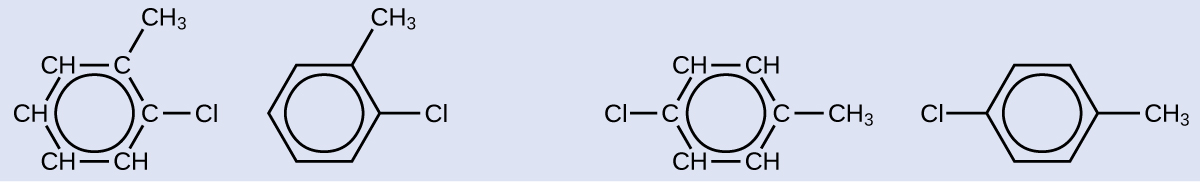# 1.4: Unsaturated Hydrocarbons

$$\newcommand{\vecs}{\overset { \rightharpoonup} {\mathbf{#1}} }$$ $$\newcommand{\vecd}{\overset{-\!-\!\rightharpoonup}{\vphantom{a}\smash {#1}}}$$$$\newcommand{\id}{\mathrm{id}}$$ $$\newcommand{\Span}{\mathrm{span}}$$ $$\newcommand{\kernel}{\mathrm{null}\,}$$ $$\newcommand{\range}{\mathrm{range}\,}$$ $$\newcommand{\RealPart}{\mathrm{Re}}$$ $$\newcommand{\ImaginaryPart}{\mathrm{Im}}$$ $$\newcommand{\Argument}{\mathrm{Arg}}$$ $$\newcommand{\norm}{\| #1 \|}$$ $$\newcommand{\inner}{\langle #1, #2 \rangle}$$ $$\newcommand{\Span}{\mathrm{span}}$$ $$\newcommand{\id}{\mathrm{id}}$$ $$\newcommand{\Span}{\mathrm{span}}$$ $$\newcommand{\kernel}{\mathrm{null}\,}$$ $$\newcommand{\range}{\mathrm{range}\,}$$ $$\newcommand{\RealPart}{\mathrm{Re}}$$ $$\newcommand{\ImaginaryPart}{\mathrm{Im}}$$ $$\newcommand{\Argument}{\mathrm{Arg}}$$ $$\newcommand{\norm}{\| #1 \|}$$ $$\newcommand{\inner}{\langle #1, #2 \rangle}$$ $$\newcommand{\Span}{\mathrm{span}}$$$$\newcommand{\AA}{\unicode[.8,0]{x212B}}$$

##### Learning Objectives
• To name alkenes given formulas and write formulas for alkenes given names.

Alkenes are hydrocarbons with carbon-to-carbon double bonds (R2C=CR2) and alkynes are hydrocarbons with carbon-to-carbon triple bonds (R–C≡C–R). In these condensed structural formulas "R" is an abbreviation for the rest of the molecule. R-groups are often used in organic chemistry when one distinct part of a molecule is being discussed.

Collectively, alkenes and alkynes are called unsaturated hydrocarbons because they have fewer hydrogen atoms than does an alkane with the same number of carbon atoms, as is indicated in the following general formulas:## Alkenes

Organic compounds that contain one or more double or triple bonds between carbon atoms are described as unsaturated. You have likely heard of unsaturated fats. These are complex organic molecules with long chains of carbon atoms, which contain at least one double bond between carbon atoms. Unsaturated hydrocarbon molecules that contain one or more double bonds are called alkenes. Carbon atoms linked by a double bond are bound together by two bonds, one σ bond and one π bond. Double and triple bonds give rise to a different geometry around the carbon atom that participates in them, leading to important differences in molecular shape and properties. The differing geometries are responsible for the different properties of unsaturated versus saturated fats.

Ethene, C2H4, is the simplest alkene. Each carbon atom in ethene, commonly called ethylene, has a trigonal planar structure. The second member of the series is propene (propylene) (Figure $$\PageIndex{1}$$); the butene isomers follow in the series. Four carbon atoms in the chain of butene allows for the formation of isomers based on the position of the double bond, as well as a new form of isomerism.Figure $$\PageIndex{1}$$: Expanded structures, ball-and-stick structures, and space-filling models for the alkenes ethene, propene, and 1-butene are shown.

Some representative alkenes—their names, structures, and physical properties—are given in Table $$\PageIndex{1}$$.

Table $$\PageIndex{1}$$: Physical Properties of Some Selected Alkenes
IUPAC Name Molecular Formula Condensed Structural Formula Melting Point (°C) Boiling Point (°C)
ethene C2H4 CH2=CH2 –169 –104
propene C3H6 CH2=CHCH3 –185 –47
1-butene C4H8 CH2=CHCH2CH3 –185 –6
1-pentene C5H10 CH2=CH(CH2)2CH3 –138 30
1-hexene C6H12 CH2=CH(CH2)3CH3 –140 63
1-heptene C7H14 CH2=CH(CH2)4CH3 –119 94
1-octene C8H16 CH2=CH(CH2)5CH3 –102 121

The first two alkenes in Table $$\PageIndex{1}$$, ethene and propene, are most often called by their common names—ethylene and propylene, respectively (Figure $$\PageIndex{2}$$). Ethylene is a major commercial chemical. The US chemical industry produces about 25 billion kilograms of ethylene annually, more than any other synthetic organic chemical. More than half of this ethylene goes into the manufacture of polyethylene, one of the most familiar plastics. Propylene is also an important industrial chemical. It is converted to plastics, isopropyl alcohol, and a variety of other products.Figure $$\PageIndex{2}$$: Ethene and Propene. The ball-and-spring models of ethene/ethylene (a) and propene/propylene (b) show their respective shapes, especially bond angles.

Although there is only one alkene with the formula C2H4 (ethene) and only one with the formula C3H6 (propene), there are several alkenes with the formula C4H8.

Here are some basic rules for naming alkenes from the International Union of Pure and Applied Chemistry (IUPAC):

1. The longest chain of carbon atoms containing the double bond is considered the parent chain. It is named using the same stem as the alkane having the same number of carbon atoms but ends in -ene to identify it as an alkene. Thus the compound CH2=CHCH3 is propene.
2. If there are four or more carbon atoms in a chain, we must indicate the position of the double bond. The carbons atoms are numbered so that the first of the two that are doubly bonded is given the lower of the two possible numbers.The compound CH3CH=CHCH2CH3, for example, has the double bond between the second and third carbon atoms. Its name is 2-pentene (not 3-pentene).
3. Substituent groups are named as with alkanes, and their position is indicated by a number. Thus, the structure below is 5-methyl-2-hexene. Note that the numbering of the parent chain is always done in such a way as to give the double bond the lowest number, even if that causes a substituent to have a higher number. The double bond always has priority in numbering.##### Example $$\PageIndex{1}$$

Name each compound.

1.1.Solution

1. The longest chain containing the double bond has five carbon atoms, so the compound is a pentene (rule 1). To give the first carbon atom of the double bond the lowest number (rule 2), we number from the left, so the compound is a 2-pentene. There is a methyl group on the fourth carbon atom (rule 3), so the compound’s name is 4-methyl-2-pentene.
2. The longest chain containing the double bond has five carbon atoms, so the parent compound is a pentene (rule 1). To give the first carbon atom of the double bond the lowest number (rule 2), we number from the left, so the compound is a 2-pentene. There is a methyl group on the third carbon atom (rule 3), so the compound’s name is 3-methyl-2-pentene.
##### Exercise $$\PageIndex{1}$$

Name each compound.

1. CH3CH2CH2CH2CH2CH=CHCH3

2.Just as there are cycloalkanes, there are cycloalkenes. These compounds are named like alkenes, but with the prefix cyclo- attached to the beginning of the parent alkene name.

##### Example $$\PageIndex{2}$$

Draw the structure for each compound.

1. 3-methyl-2-pentene
2. cyclohexene

Solution

1. First write the parent chain of five carbon atoms: C–C–C–C–C. Then add the double bond between the second and third carbon atoms:Now place the methyl group on the third carbon atom and add enough hydrogen atoms to give each carbon atom a total of four bonds.2. First, consider what each of the three parts of the name means. Cyclo means a ring compound, hex means 6 carbon atoms, and -ene means a double bond.##### Exercise $$\PageIndex{2}$$

Draw the structure for each compound.

1. 2-ethyl-1-hexene
2. cyclopentene

## Alkynes

Hydrocarbon molecules with one or more triple bonds are called alkynes; they make up another series of unsaturated hydrocarbons. Two carbon atoms joined by a triple bond are bound together by one σ bond and two π bonds. The sp-hybridized carbons involved in the triple bond have bond angles of 180°, giving these types of bonds a linear, rod-like shape.

The simplest member of the alkyne series is ethyne, C2H2, commonly called acetylene. The Lewis structure for ethyne, a linear molecule, is:The IUPAC nomenclature for alkynes is similar to that for alkenes except that the suffix -yne is used to indicate a triple bond in the chain. For example, $$\mathrm{CH_3CH_2C≡CH}$$ is called 1-butyne.

##### Example $$\PageIndex{3}$$: Structure of Alkynes

Describe the geometry and hybridization of the carbon atoms in the following molecule:Solution

Carbon atoms 1 and 4 have four single bonds and are thus tetrahedral with sp3 hybridization. Carbon atoms 2 and 3 are involved in the triple bond, so they have linear geometries and would be classified as sp hybrids.

##### Exercise $$\PageIndex{3}$$

Identify the hybridization and bond angles at the carbon atoms in the molecule shown:carbon 1: sp, 180°; carbon 2: sp, 180°; carbon 3: sp2, 120°; carbon 4: sp2, 120°; carbon 5: sp3, 109.5°

## Aromatic Hydrocarbons

Benzene, C6H6, is the simplest member of a large family of hydrocarbons, called aromatic hydrocarbons. These compounds contain ring structures and exhibit bonding that must be described using the resonance hybrid concept of valence bond theory or the delocalization concept of molecular orbital theory. (To review these concepts, refer to the earlier chapters on chemical bonding). The resonance structures for benzene, C6H6, are:Figure $$\PageIndex{3}$$.Figure $$\PageIndex{3}$$: This condensed formula shows the unique bonding structure of benzene.

There are many derivatives of benzene. The hydrogen atoms can be replaced by many different substituents. Aromatic compounds more readily undergo substitution reactions than addition reactions; replacement of one of the hydrogen atoms with another substituent will leave the delocalized double bonds intact. The following are typical examples of substituted benzene derivatives:Toluene and xylene are important solvents and raw materials in the chemical industry. Styrene is used to produce the polymer polystyrene.

##### Example $$\PageIndex{4}$$: Structure of Aromatic Hydrocarbons

One possible isomer created by a substitution reaction that replaces a hydrogen atom attached to the aromatic ring of toluene with a chlorine atom is shown here. Draw two other possible isomers in which the chlorine atom replaces a different hydrogen atom attached to the aromatic ring:Solution

Since the six-carbon ring with alternating double bonds is necessary for the molecule to be classified as aromatic, appropriate isomers can be produced only by changing the positions of the chloro-substituent relative to the methyl-substituent:##### Exercise $$\PageIndex{4}$$

Draw three isomers of a six-membered aromatic ring compound substituted with two bromines.